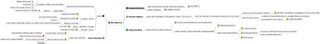## Monday, July 17, 2006

### Overview of Mathematics

Click Below Mindmap to see overview of Mathematics. Most of the details are from Wikipedia.Above mindmap classifies Maths into four field:
1. Quantity
Here we identify what number is. ie. we are dealing with real axis
This branch of Maths has to do with calculation. eg. If I buy a cake worth of Rs. 45 and give you Rs 50 note , you will have to give me Rs. 5 back .

2. Structure
This branch deals with relation between number. eg. If x=1, y=3; then x+y=4
(Assume we have 2 or more nos on same real axis interacting with each other)

3. Space
Here we bring into another axis. This branch deals with measuring land. Therefore, here basic unit is a point which is ordered pair of 2 nos.

4. Change
This relates with function that shows change.

(Please see Wikipedia for more detail)